# How to Select and Copy Cell Values from Every Nth Column in Excel

This post will guide you how to select all Cell values from every nth column in your worksheet in Excel. How do I select all cells in every nth column using a VBA Macro in Excel. How do I retrive or copy cell values from every nth column using a formula in Excel 2013/2016.

Assuming that you have a list of data with hundreds of columns in a worksheet , and you wish to retrive all cell values from every second or third or nth column, etc. How to do it. You can select all cells from every nth column using a VBA macro quickly, and then press` Ctrl + C` short cuts to copy those selected cells. or You can use a formula to retrive all cell values from every nth column based on the` OFFSET function` and the` COLUMN function`.

## Select All Cells from Every Nth Column Using VBA Macro

To select all cells from every Nth column (such as: third column), and you can use an Excel VBA macro to quickly select them with the following steps:

Step1: open your excel workbook and then click on “`Visual Basic`” command under `DEVELOPER` Tab, or just press “`ALT+F11`” shortcut.Step2: then the “`Visual Basic Editor`” window will appear.

Step3: click “`Insert`” ->”`Module`” to create a new module.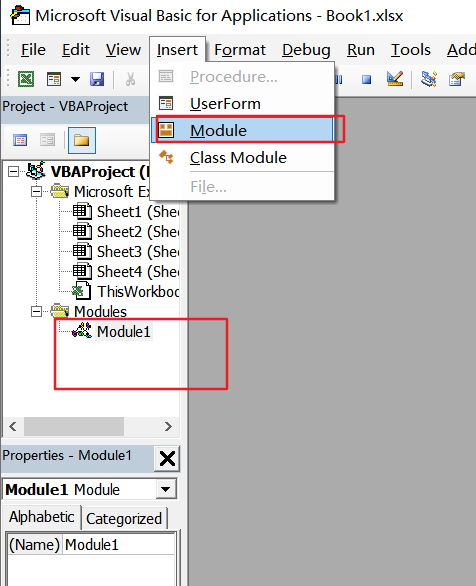Step4: paste the below VBA code  into the code window. Then clicking “`Save`” button.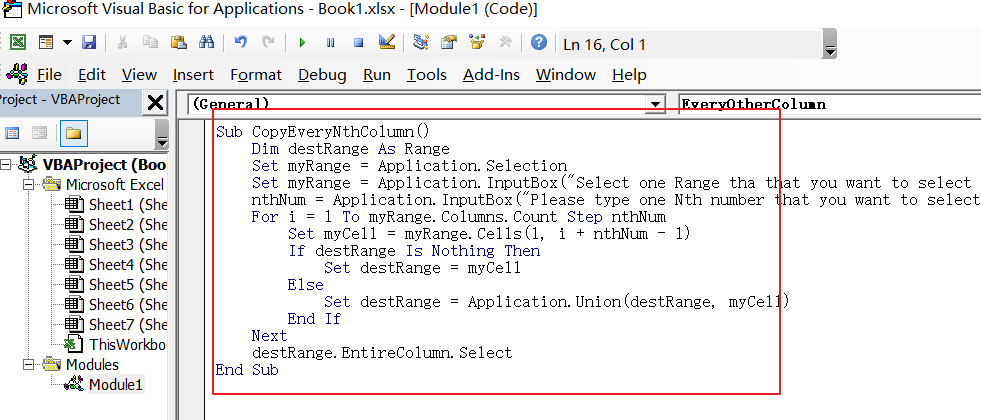```Sub CopyEveryNthColumn()
Dim destRange As Range
Set myRange = Application.Selection
Set myRange = Application.InputBox("Select one Range tha that you want to select All nth columns:", "CopyEveryNthColumn", myRange.Address, Type:=8)
nthNum = Application.InputBox("Please type one Nth number that you want to select: ", "CopyEveryNthColumn", Type:=1)
For i = 1 To myRange.Columns.Count Step nthNum
Set myCell = myRange.Cells(1, i + nthNum - 1)
If destRange Is Nothing Then
Set destRange = myCell
Else
Set destRange = Application.Union(destRange, myCell)
End If
Next
destRange.EntireColumn.Select
End Sub```

Step5: back to the current worksheet, click on `Macros` button under `Code` group. then click `Run` button.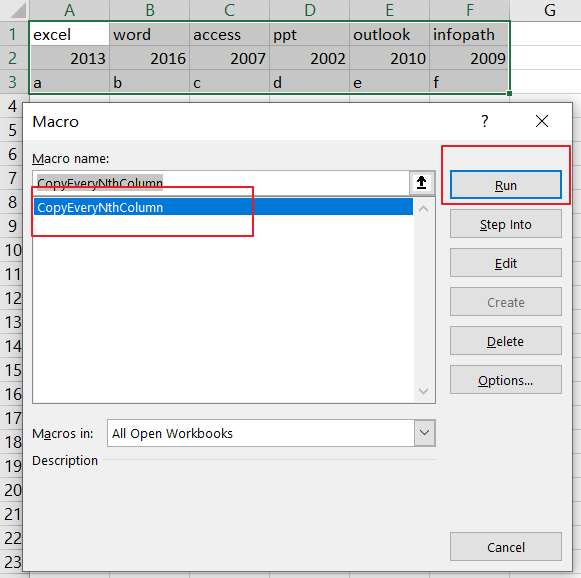Step6: Select one Range tha that you want to select All nth columns, such as: A1:F3 click on `Ok` button.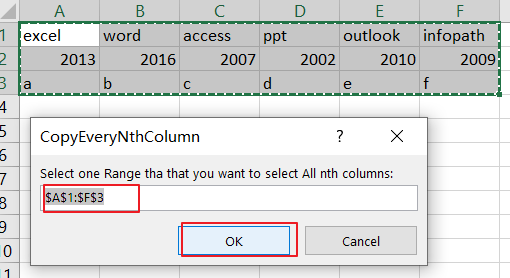Step7:Please type one Nth number that you want to select, such as: number 3. click on `Ok` button.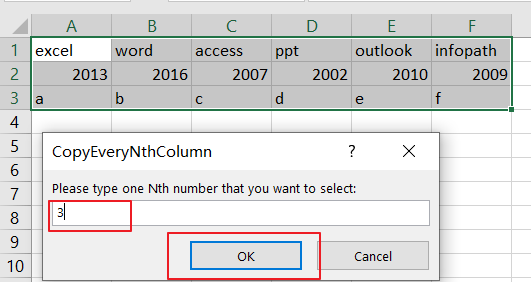Step8: you would see that every 3rd column has been selected. and then you can press Ctrl+C keys to copy them.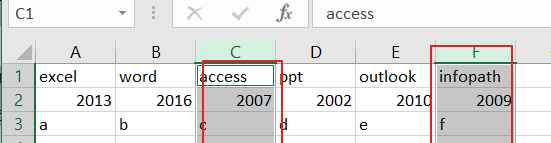## Retrive All Cell Values from Every Nth Column Using Formula

You can also use an formula to copy or retrive cell values from every nth column(third column). see below:

`=OFFSET(\$A1,0,(COLUMN(A1)*3)-1)`

You need to type this formula into a blank cell (such as: A5)and press Enter key on your keyboard to apply it. then drag the AutoFill Handle from Cell A5 to same row cells unitl number 0 is displayed.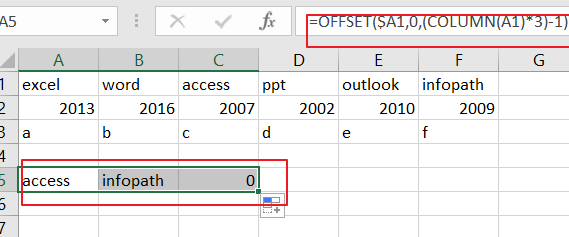Keeping cells are selected. and drag the AutoFill handle down to other cells unitl number 0 is displayed.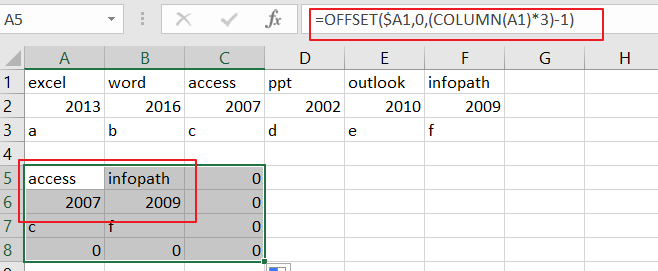Note: if you want to select or copy cell values from nth column in your worksheet, and you just need to change number 3 as you need in the above formula.

### Related Functions

• Excel COLUMN function
The Excel COLUMN function returns the first column number of the given cell reference.The syntax of the COLUMN function is as below:=COLUMN ([reference])….
Related Posts

Find and Replace Multiple Values

This post will guide you how to find and replace multiple values at once with VBA macro or using formula  in Excel. How do I make multiple find and replace in Excel. Suppose that you have a few cells containing ...

VBA Macro For VLOOKUP From Another Sheet

In the previous post, you should know that how to fix or remove the #N/A error when using VLOOKUP formula to lookup value from another sheet. And this post will show you how to use VBA code to vlookup data ...

How To Insert Comments in Protected Worksheet in Excel

This post will show you how to allow comments in a protected worksheet in Excel. You can easily to insert comments into cells in a normal worksheet in Excel, but if want to insert a comment in a worksheet that ...

How To Convert Text to Upper Cases(Using VBA) in Excel

This post will show you how to switch from lower case to upper case in Excel. and I am going to show you two different ways of converting text to upper cases using formula or VBA macro in Excel 2013,Excel ...

How To Hide Every Other Row in Excel (Using VBA)

This post will show you how to hide alternate rows or columns in Excel or how to hide every third, fourth, fifth row or column in Excel. If you want to hide every other row in your current worksheet, how ...

How to Disable the Save As Prompt in Excel

This post will show you how to use a VBA Macro to save an Excel file and overwrite any existing file without a prompt so that you are going to get the little window that says file already exists do ...

How to Count Cells that Contain even or odd numbers in Excel

This post will guide you how to count the number of cells that contain odd or even numbers within a range of cells using a formula in Excel 2013/2016.How do I count cells that contain odd numbers through the use ...

How to Count Cells that Contain negative Numbers in Excel

This post will guide you how to count the number of cells that contain negative numbers within a range of cells using a formula in Excel 2013/2016.You can count the number of negative numbers in your data using easy functions ...

How to Count Cells Are Not Blank or Empty in Excel

This post will guide you how to count cells that are not blank or empty in a given range cells using a formula in Excel 2013/2016.How do I count the number of cells that are not blank in a particular ...

How to Count Cells Less Than a Specific Value in Excel

This post will guide you how to count the number of cells less than a particular numeric value in a given range cells using a formula in Excel 2013/2016. How do I count cells that are less than a specific ...

Sidebar